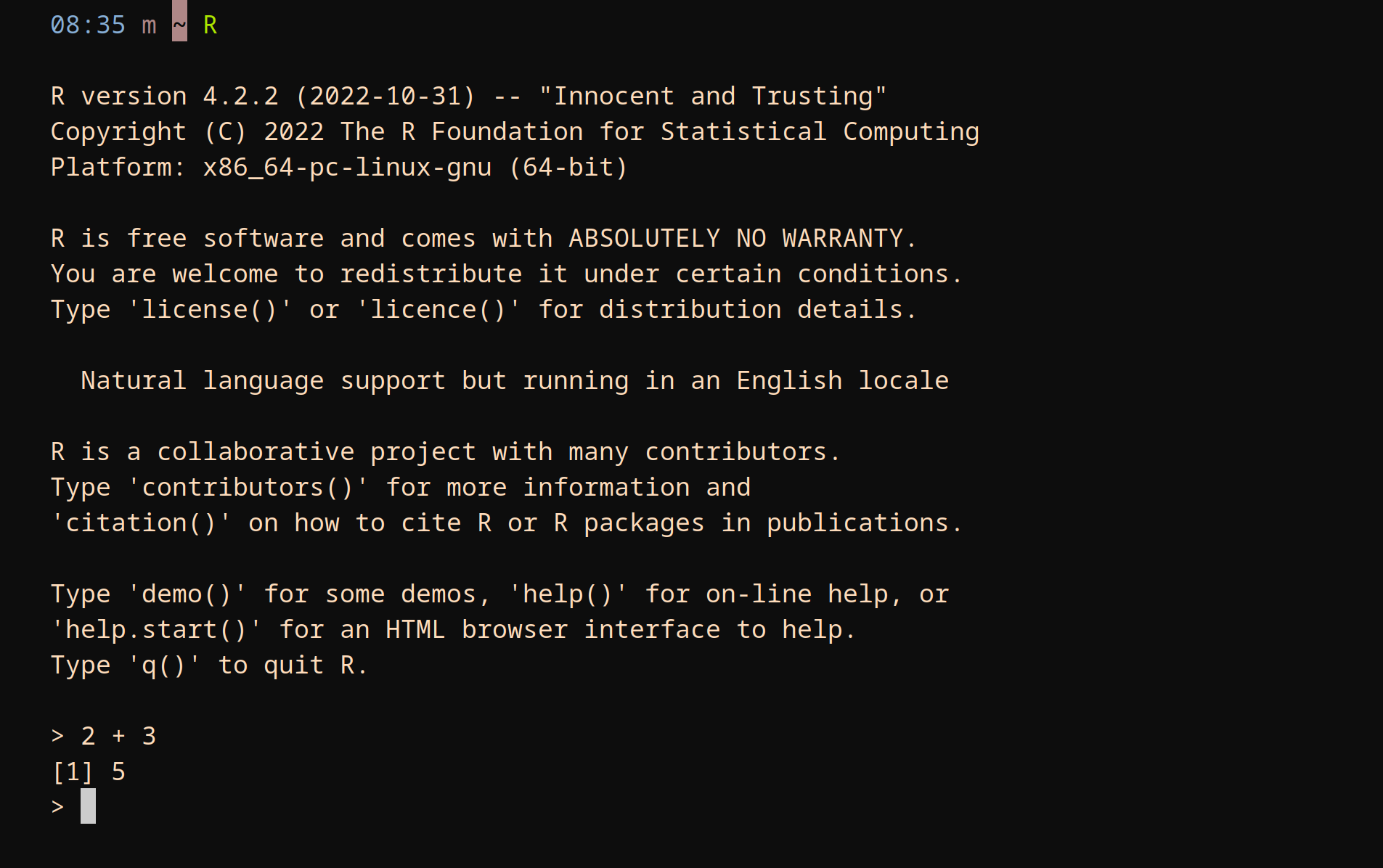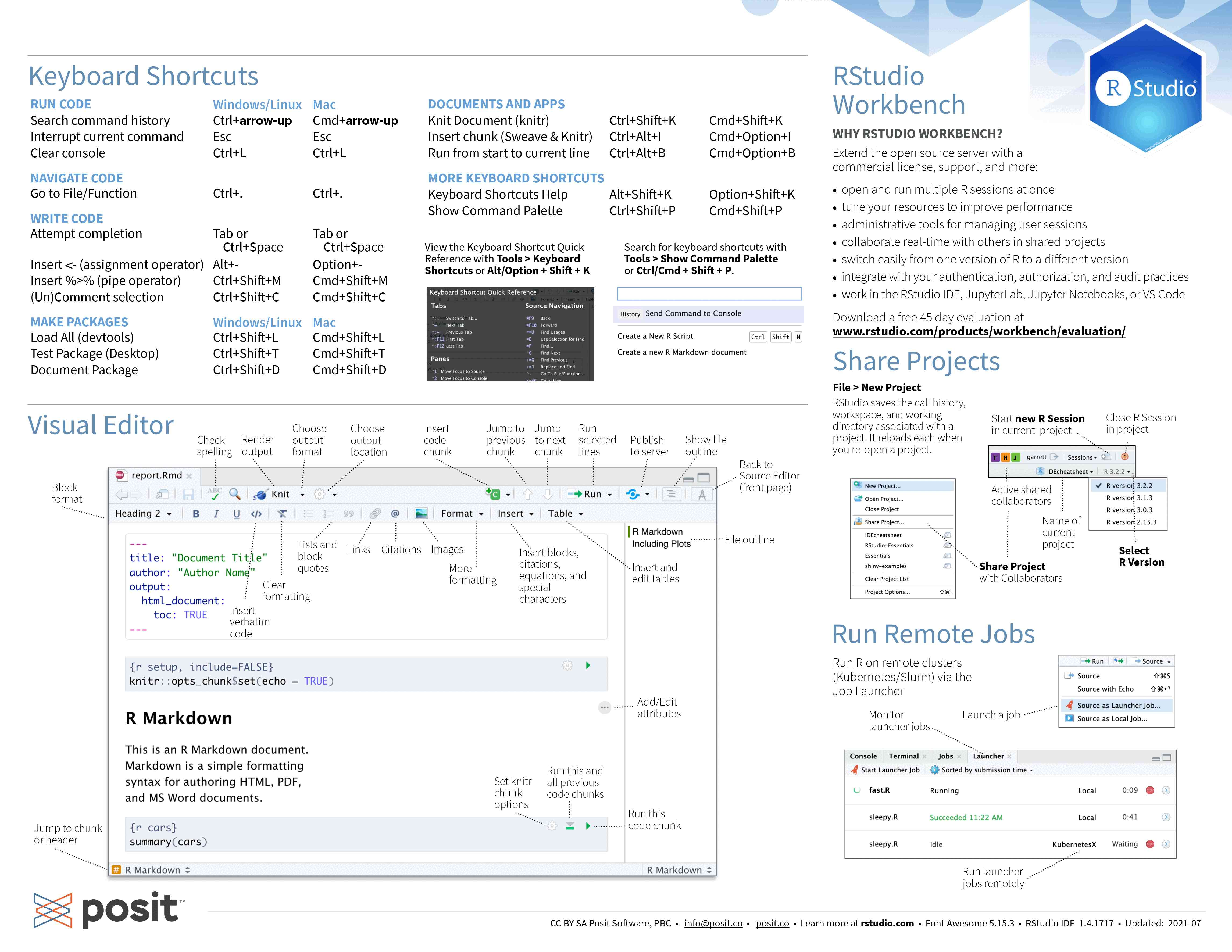# Introduction to R for the humanities

Author

Marie-Hélène Burle

R is a free and open-source programming language for statistical computing, modelling, and graphics, with an unbeatable collection of statistical packages. It is extremely popular in some academic fields such as statistics, biology, bioinformatics, data mining, data analysis, and linguistics.

This introductory course does not assume any prior knowledge.

## Running R

R being an interpreted language, it can be run non-interactively or interactively.

### Running R non-interactively

If you write code in a text file (called a script), you can then execute it with:

``Rscript my_script.R``

The command to execute scripts is `Rscript` rather than `R`.
By convention, R scripts take the extension `.R`.

### Running R interactively

There are several ways to run R interactively.

• Directly in the console (the name for the R shell):The RStudio IDE is popular and this is what we will use today. RStudio can can be run locally, but for this course, we will use an RStudio server.

### Accessing our RStudio server

For this workshop, we will use a temporary RStudio server.

To access it, go to the website given during the workshop and sign in using the username and password you will be given (you can ignore the OTP entry).

This will take you to our JupyterHub. There, click on the “RStudio” button and our RStudio server will open in a new tab.

### Using RStudio

For those unfamiliar with the RStudio IDE, you can download the following cheatsheet:## Help and documentation

For some general documentation on R, you can run:

``help.start()``

To get help on a function (e.g. `sum`), you can run:

``help(sum)``

Depending on your settings, this will open a documentation for `sum` in a pager or in your browser.

## Basic syntax

### Assignment

R can accept the equal sign (`=`) for assignments, but it is more idiomatic to use the assignment sign (`<-`) whenever you bind a name to a value and to use the equal sign everywhere else.

``a <- 3``

Once you have bound a name to a value, you can recall the value with that name:

``a  # Note that you do not need to use a print() function in R``
`` 3``

You can remove an object from the environment by deleting its name:

``````rm(a)
a``````
``Error in eval(expr, envir, enclos): object 'a' not found``

The garbage collector will take care of deleting the object itself from memory.

Anything to the left of `#` is a comment and is ignored by R:

``````# This is an inline comment

a <- 3  # This is also a comment``````

## Data types and structures

Dimension Homogeneous Heterogeneous
1 d Atomic vector List
2 d Matrix Data frame
3 d Array

### Atomic vectors

``````vec <- c(2, 4, 1)
vec``````
`` 2 4 1``
``typeof(vec)``
`` "double"``
``str(vec)``
`` num [1:3] 2 4 1``
``````vec <- c(TRUE, TRUE, NA, FALSE)
vec``````
``  TRUE  TRUE    NA FALSE``
``typeof(vec)``
`` "logical"``
``str(vec)``
`` logi [1:4] TRUE TRUE NA FALSE``

`NA` (“Not Available”) is a logical constant of length one. It is an indicator for a missing value.

Vectors are homogeneous, so all elements need to be of the same type.

If you use elements of different types, R will convert some of them to ensure that they become of the same type:

``````vec <- c("This is a string", 3, "test")
vec``````
`` "This is a string" "3"                "test"            ``
``typeof(vec)``
`` "character"``
``str(vec)``
`` chr [1:3] "This is a string" "3" "test"``
``````vec <- c(TRUE, 3, FALSE)
vec``````
`` 1 3 0``
``typeof(vec)``
`` "double"``
``str(vec)``
`` num [1:3] 1 3 0``

### Data frames

Data frames contain tabular data. Under the hood, a data frame is a list of vectors.

``````dat <- data.frame(
var = c(2.9, 3.1, 4.5)
)
dat``````
``````  country var
2     USA 3.1
3  Mexico 4.5``````
``typeof(dat)``
`` "list"``
``str(dat)``
``````'data.frame':   3 obs. of  2 variables:
\$ country: chr  "Canada" "USA" "Mexico"
\$ var    : num  2.9 3.1 4.5``````
``length(dat)``
`` 2``
``dim(dat)``
`` 3 2``

## Function definition

``````compare <- function(x, y) {
x == y
}``````

We can now use our function:

``compare(2, 3)``
`` FALSE``

Note that the result of the last statement is printed automatically:

``````test <- function(x, y) {
x
y
}
test(2, 3)``````
`` 3``

If you want to return other results, you need to explicitly use the `print()` function:

``````test <- function(x, y) {
print(x)
y
}
test(2, 3)``````
`` 2``
`` 3``

## Control flow

### Conditionals

``````test_sign <- function(x) {
if (x > 0) {
"x is positif"
} else if (x < 0) {
"x is negatif"
} else {
"x is equal to zero"
}
}``````
``test_sign(3)``
`` "x is positif"``
``test_sign(-2)``
`` "x is negatif"``
``test_sign(0)``
`` "x is equal to zero"``

### Loops

``````for (i in 1:10) {
print(i)
}``````
`````` 1
 2
 3
 4
 5
 6
 7
 8
 9
 10``````

Notice that here we need to use the `print()` function.

## Packages

Packages are a set of functions and/or data that add functionality to R.

### Managing R packages

R packages can be installed, updated, and removed from within R:

``````install.packages("package-name")
remove.packages("package-name")
update_packages()``````

To make a package available in an R session, you load it with the `library()` function.

Example:

``library(readxl)``

Alternatively, you can access a function from a package without loading it with the syntax: `package::function()`.

Example:

``readxl::read_excel("file.xlsx")``

## Publishing

You might have heard of R Markdown. It allows for the creation of dynamic publication-quality documents mixing code blocks, text, graphs…

The team which created R Markdown has now created an even better tool: Quarto. If you are interested in an introduction to this tool, you can have a look at our workshop or our webinar on Quarto.

First part

Second part